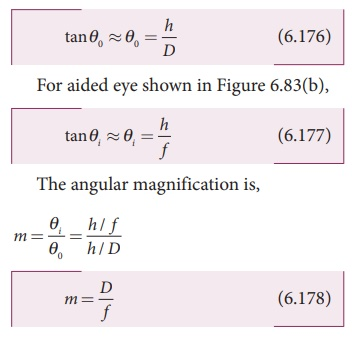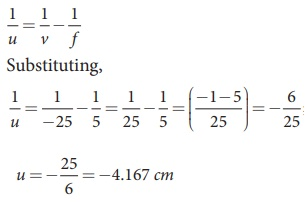Home | | Physics 12th Std | Simple microscope

# Simple microscope

A simple microscope is a single magnifying (converging) lens of small focal length. Magnification in normal focusing (angular magnification), Resolving power of microscope

Simple microscope

A simple microscope is a single magnifying (converging) lens of small focal length. The idea is to get an erect, magnified and virtual image of the object. For this the object is placed between F and P on one side of the lens and viewed from other side of the lens. There are two magnifications to be discussed for two kinds of focussing.

(1) Near point focusing – The image is formed at near point, i.e. 25 cm for normal eye. This distance is also called as least distance D of distinct vision. In this position, the eye feels comfortable but there is little strain on the eye. This is shown in Figure 6.83

(2) Normal focusing – The image is formed at infinity. In this position the eye is most relaxed to view the image. This is shown in Figure 6.84(b).

## Magnification in near point focusing

The near point focusing is shown in Figure 6.83. Object distance u is less than f. The image distance is the near point D. The magnification m is given by the relation,With the help of lens equation, 1/v – 1/u = 1/f  the magnification can further be written as,Substituting for v with sign convention, v = –DThis is the magnification for near point focusing.## Magnification in normal focusing (angular magnification)

The normal focusing is shown in Figure 6.84(b). We will now find the magnification for the image formed at infinity. If we take the ratio of height of image to height of object (m=h’/h) to find the magnification, we will not get a practical relation, as the image will also be of infinite size when the image is formed at infinity. Hence, we can practically use the angular magnification. The angular magnification is defined as the ratio of angle θi subtended by the image with aided eye to the angle θ0 subtended by the object with unaided eye.For unaided eye shown in Figure 6.84(a),This is the magnification for normal focusing.

The magnification for normal focusing is one less than that for near point focusing. But, the viewing is more comfortable in normal focusing than near point focusing. For large values of D/f, the difference in magnification is usually small. In subsequent discussions, we shall only consider the normal focusing.

### EXAMPLE 6.41

A man with a near point of 25 cm reads a book with small print using a magnifying glass, a convex lens of focal length 5 cm. (a) What is the closest and the farthest distance at which he should keep the lens from the page so that he can read the book when viewing through the magnifying glass? (b) What is the maximum and the minimum angular magnification (magnifying power) possible using the above simple microscope?

### Solution

D = 25 cm; f = 5 cm;

For closest object distance, u; the image distance, v is, –25 cm. (near point focusing)

For farthest object distance, u’; the corresponding image distance, v’ is, v’ = ∞ (normal focusing)

(a) To find closest image distance, lens equation, 1/v – 1/u = 1/f

Rewriting for closest object distance, 1/u = 1/v - 1/fThe closest distance at which the person should can keep the book is, u = –4.167 cm

To find farthest object distance, lens equation is, 1/v’ – 1/u’ = 1/f’

Rewriting for farthest object distance, 1/u’ = 1/v’ - 1/f’

Substituting, 1/u’ = 1/∞ - 1/5; u = −5 cm

The farthest distance at which the person can keep the book is, u′ = −5 cm

(b) To find magnification in near point focusing, m = 1+ D/f = 1+ 25/5 = 6

To find magnification in normal focusing,

m = D/f = 25/5 = 5

## Resolving power of microscope

The diagram related to the calculation of resolution of microscope is illustrated in Figure 6.85. A microscope is used to see the details of the object under observation. The ability of microscope depends not only in magnifying the object but also in resolving two points on the object separated by a small distance dmin. Smaller the value of dmin better will be the resolving power of the microscope.In the place of focal length f we have the image distance v. If the difference between the two points on the object to be resolved is dmin, then the magnification m is,To further reduce the value of dmin the optical path of the light is increased by immersing the objective of the microscope in to a bath containing oil of refractive index n.Such an objective is called oil immersed objective. The term nsinβ is called numerical aperture NA.Tags : Near point focusing, Normal focusing | Optical Instruments , 12th Physics : UNIT 7 : Wave Optics
Study Material, Lecturing Notes, Assignment, Reference, Wiki description explanation, brief detail
12th Physics : UNIT 7 : Wave Optics : Simple microscope | Near point focusing, Normal focusing | Optical Instruments